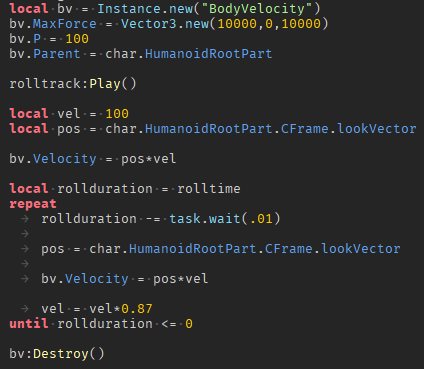# Bodyvelocity goes faster in certain directions?!?!

https://gyazo.com/69cb95511655f8e119b4fb2f872d125c

I have a roll script using body velocities inside of the players humanoidrootpart for some reason its faster in some directions. Why is this?

try looking at max force. it almost definitely causes this

2 Likes

max force is 10000,0,10000 I dont think it does but maybe im wrong.

1 Like

If in any case the angles are equal to or close to 45 degrees, it could be explained in this way:
`c = (x + z) * p`

`p` Is the impulse

1 Like

You need to normalize the direction of the vector before you apply it to a velocity.

To do that simply use
`Direction.Unit`
Example:

``````local Direction = Humanoid.MoveDirection.Unit
local Speed = 10
BodyVelocity.Velocity = Speed * Direction
``````

If you are using the MoveDirection of the humanoid or are taking raw input, you will need to normalize it so that the length of the direction remains the same length of 1

2 Likes

I do something very similar already.

I use the lookvector of the cframe of the humanoidrootpart and times it by 100

Could you show us the code please?

1 LikeI still have no clue why this is happening.

This could be a roblox bug of some sort or something. I have a very similar system in another game and I don’t have this problem.

Have you looked into this?

You can see if this is the problem by setting the MaxForce to a much larger number and seeing if that removes the speed difference. I’d recommend setting the max force such that increasing it more doesn’t increase the resulting speed.

Edit:

Yep! Some of the math was a bit off, though it’s the right idea.

The max force on the x axis is `MaxForce.X` and the max force on the z axis is `MaxForce.Z`. The magnitude on a single axis is either `MaxForce.X` or `MaxForce.Z`, but at any direction that’s not directly on the z or x axis would form a right triangle with the `MaxForce.X` and `MaxForce.Z`.

So like, MaxForce = Vector3.new(1,0,1) would have a magnitude of 1 if the direction is going on the x or z, but at a 45 degree angle from both the x and z axis it would form a longer-line/right-triangle between the two, which has a greater magnitude:My drawing isn’t perfect, but notice how the magnitude at 45 degrees is longer than the other ones (when the x and z axes are capped at one).

2 Likes

ok now even what i put confuses meif i understand, you are saying that the equation I have made explains the problem in a certain way

1 Like